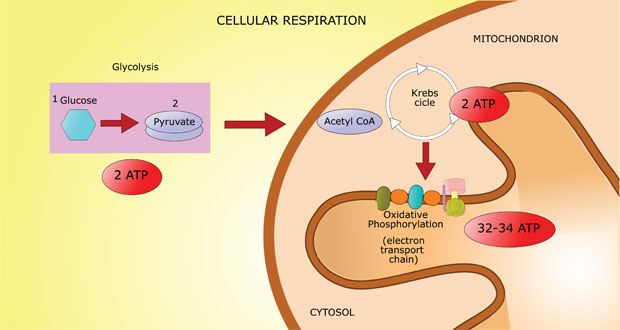# Cellular RespirationWhat is cellular respiration? Cellular Respiration is the process that takes place in cells to convert food into energy. This process is also known as internal respiration. In order to release the maximal amount of energy, the molecules of Carbon, Hydrogen, Oxygen, and Nitrogen which make up our food are stored as a high energy molecule known as ATP or Adenosine Triphosphate.

## Cellular respiration equation

When energy is needed, ATP is broken down using an enzyme (known as ATPase) into ADP (Adenosine Diphosphate). This process breaks the high energy Phosphate (P) bond and so provides energy for use by the body. Here is the equation you may see in textbooks:

ATP = ADP + P +Energy

The human body is also capable of resynthesising ATP to allow it to continue producing energy. To do this it must use energy to reverse the equation shown above. This is known as an endothermic reaction as it requires energy. The breakdown of ATP is called exothermic as it produces energy. The process of breaking down and resynthesising ATP is efficient at producing energy as less energy is required to resynthesis the ATP that is made to break it down. Here is the Resynthesis equation:

Energy + ADP +P = ATP

## What are the Energy Systems?

There are two energy systems used during the process of respiration.

### Aerobic respiration

Aerobic Respiration, meaning ‘with oxygen’ is used for long-term, steady-paced exercise, and day-to-day activities.

### Anaerobic respiration

Anaerobic Respiration or ‘without oxygen’ which produces fast bursts of energy for short, powerful bursts. The Anaerobic system can be divided into two further systems:

• ATP-PC
• Lactic acid

All energy systems work together, but the intensity and type of activity will determine which system is predominant.